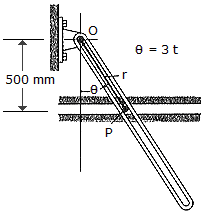# Engineering Mechanics - Kinematics of Particle (KOP) - Discussion

### Discussion :: Kinematics of Particle (KOP) - General Questions (Q.No.16)

16.The slotted link is pinned at O, and as a result of rotation it drives the peg P along the horizontal guide. Compute the magnitude of the velocity and acceleration of P along the horizontal guide. Compute the magnitudes of the velocity and acceleration of P as a function ofif= (3t) rad, where t is measured in seconds.

 [A]. v = 0.500 sec2m/s, a = 1.000 sec2tanm/s2 [B]. v = 1.500 secm/s, a = 9.00 sectanm/s2 [C]. v = 1.500 sectanm/s, a = 9.00 sectanm/s2 [D]. v = 1.500 sec2m/s, a = 9.00 sec2tanm/s2

Explanation:

No answer description available for this question.

 Fsar said: (Feb 4, 2011) How do you get to the final answer?

 Mohamed said: (Jun 17, 2021) Give the explanation of the answer.